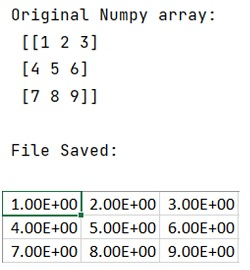# Convert a NumPy array into a CSV file

NumPy array to CSV: In this tutorial, we will learn how to convert a NumPy array into a CSV file? By Pranit Sharma Last updated : May 23, 2023

## What is NumPy array?

NumPy is an abbreviated form of Numerical Python. It is used for different types of scientific operations in python. Numpy is a vast library in python which is used for almost every kind of scientific or mathematical operation. It is itself an array which is a collection of various methods and functions for processing the arrays.

## What is a CSV file?

CSV files or Comma Separated Values files are plain text files but the format of CSV files is tabular. As the name suggests, in a CSV file, each specific value inside the CSV file is generally separated with a comma. The first line identifies the name of a data column. The further subsequent lines identify the values in rows.

Col_1_value, col_2_value , col_3_value
Row1_value1 , row_1_value2 , row_1_value3
Row1_value1 , row_1_value2 , row_1_value3

Here, the separator character (,) is called a delimiter. There are some more popular delimiters. E.g.: tab(\t), colon (:), semi-colon (;) etc.

## How to Convert a NumPy array into a CSV file?

To convert a NumPy array to a CSV file, you can use numpy.savetxt() method by passing the CSV file name, input array, and delimiter. The method saves the given NumPy array to a CSV file. Consider the following code snippet for this conversion:

```np.savetxt("arr.csv", arr, delimiter=",")
```

Let us understand with the help of an example,

## Example to Convert a NumPy array into a CSV file

```# Import numpy
import numpy as np

# Creating a Numpy arrays
arr = np.asarray([ [1,2,3], [4,5,6], [7,8,9] ])

# Display original numpy array
print("Original Numpy array:\n",arr,"\n")

# Dumping this array into a csv file
np.savetxt("arr.csv", arr, delimiter=",")

# Display a msg
print("File Saved:\n")
```

### Output